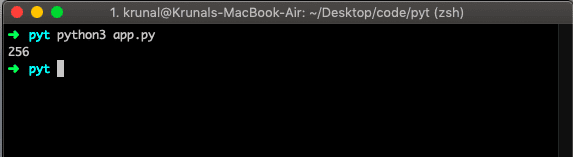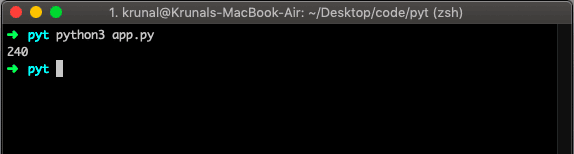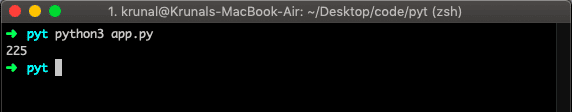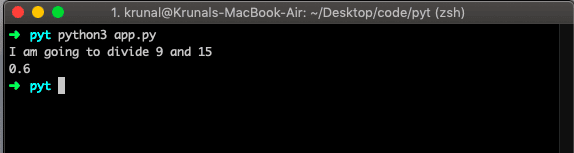# Python Decorators: The Complete Guide

In Python, everything is an object from class to function. In Python, the names we define are the identifiers bound to these objects. Functions are no exceptions to that fact because they are objects too with attributes. Therefore, in Python, Functions are first-class objects, which means the following.

1. Functions are objects; they can be referenced to another function, passed to a variable, and returned from other functions.
2. Functions can be defined inside another function and passed as an argument to another function.

Decorators provide the simple syntax for calling higher-order functions.

## Python Decorators

Decorator in Python, a decorator, is a function that takes another function and extends its behavior without explicitly changing it. The decorators are a powerful and useful feature since it allows programmers to modify the behavior of a function or class.

Let us take a simple function and then extend its behavior to make a decorator.

```# app.py

def square(x):
return x * x
print(square(16))```

The above function returns merely the square of the passing argument as a number.

See the output in the console.Now, let us extends its behavior. First, let us create a Decorator. Then, write the following code inside the app.py file.

```# app.py

def square(x):
return x * x

def decorator(func, x):
output = func(x)
output = output - x
return output

print(decorator(square, 16))```

In the above example, we have created one more function called a decorator. You can name it anything.

Then, I passed two arguments, one of the square() function and the other is the number that we need the square of.

Then, we get the square output and use that output further to perform a further calculation. For example, we can get the following output from the above decorator.## The function can return another function.

Let us take an example where a function returns another function.

```# app.py

def decorator():
def square(x):
return x * x
return square(15)

func = decorator()
print(func)```

In the above example, we call the decorator() function, which returns another function called square().

Functions and methods are called callable because they can be called.

Any object that implements the particular method called __call()__ is termed callable. So, in the most basic sense, the decorator is the callable that returns a callable.

Without calling a function, a function will not be executed. That is why we call that square() function from the decorator() function, and finally, we get the output. The output is the following.## Decorating Functions in Python

Let us take the following example.

```# app.py

def smart_divide(func):
def inner(a,b):
print("I am going to divide",a,"and",b)
if b == 0:
print("Whoops! cannot divide")
return

return func(a,b)
return inner

@smart_divide
def divide(a,b):
return a/b

print(divide(9, 15))
```

In the above example, we are dividing one number by another number. Here one gotcha is that the second number should not be zero.

That is why we are extending its functionality by adding a logic that if the second parameter is 0, we should be returned from the function and not perform the division.

That is why when we call the divide function just above, we have written the @smart_divide decorator. That means we are using smart division and not regular division.

It extends the functionality of simple division. The output of the above decorator is the following.That’s it for this tutorial.

### 1 thought on “Python Decorators: The Complete Guide”

1.Thanks for the guide